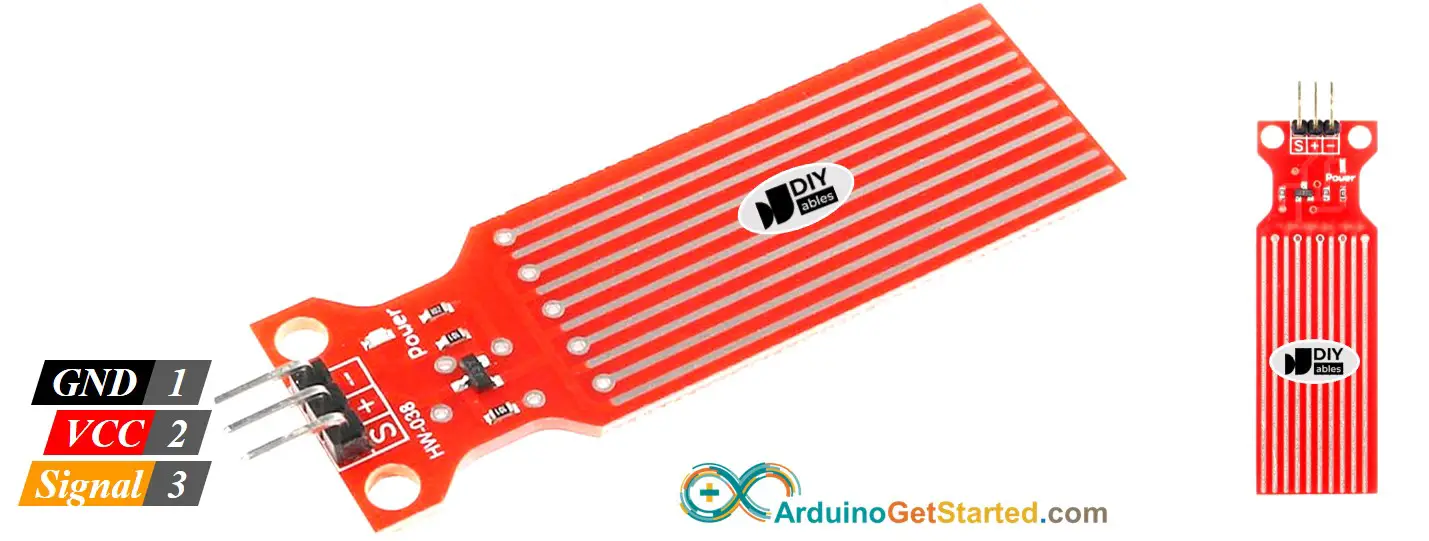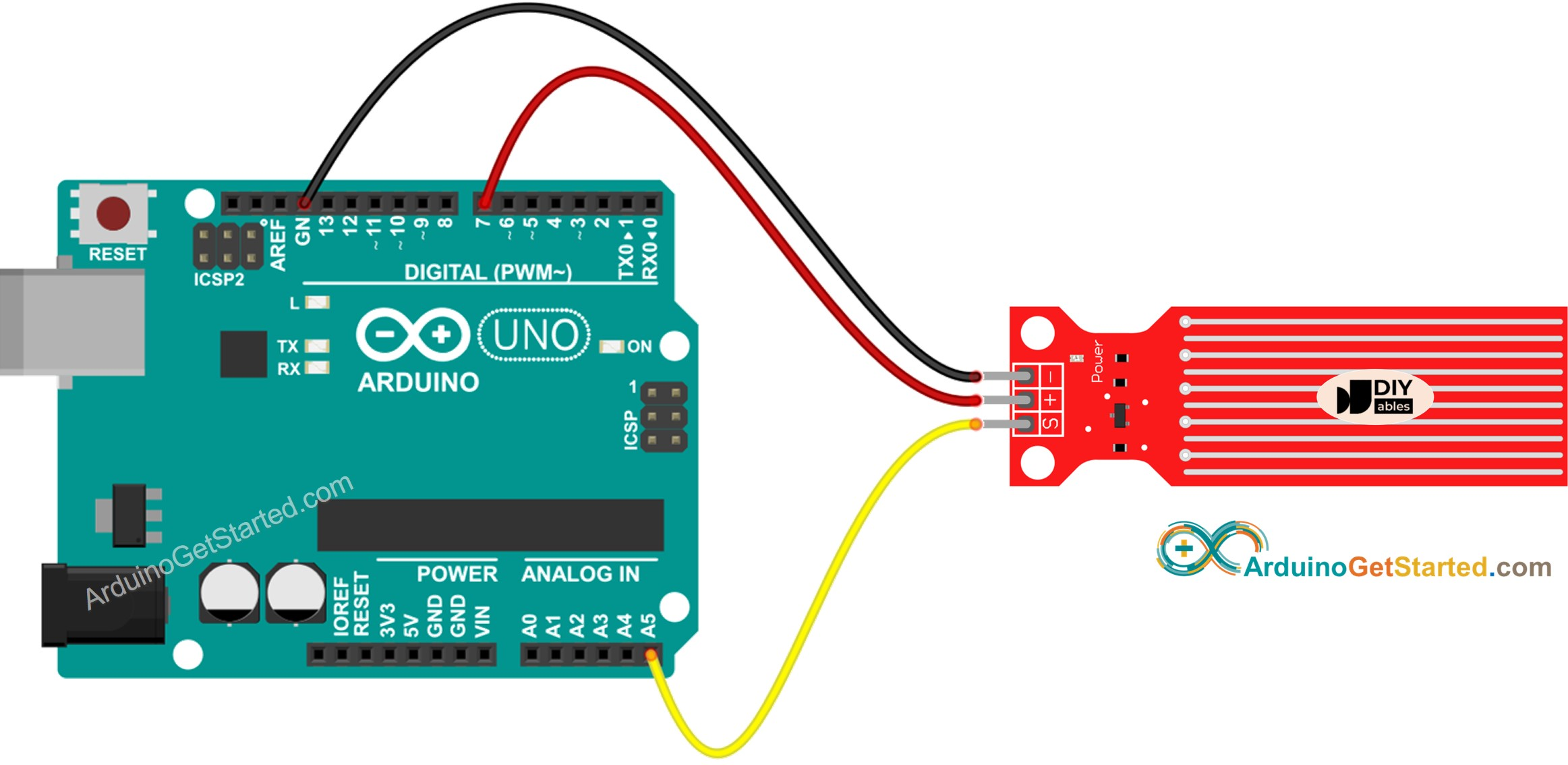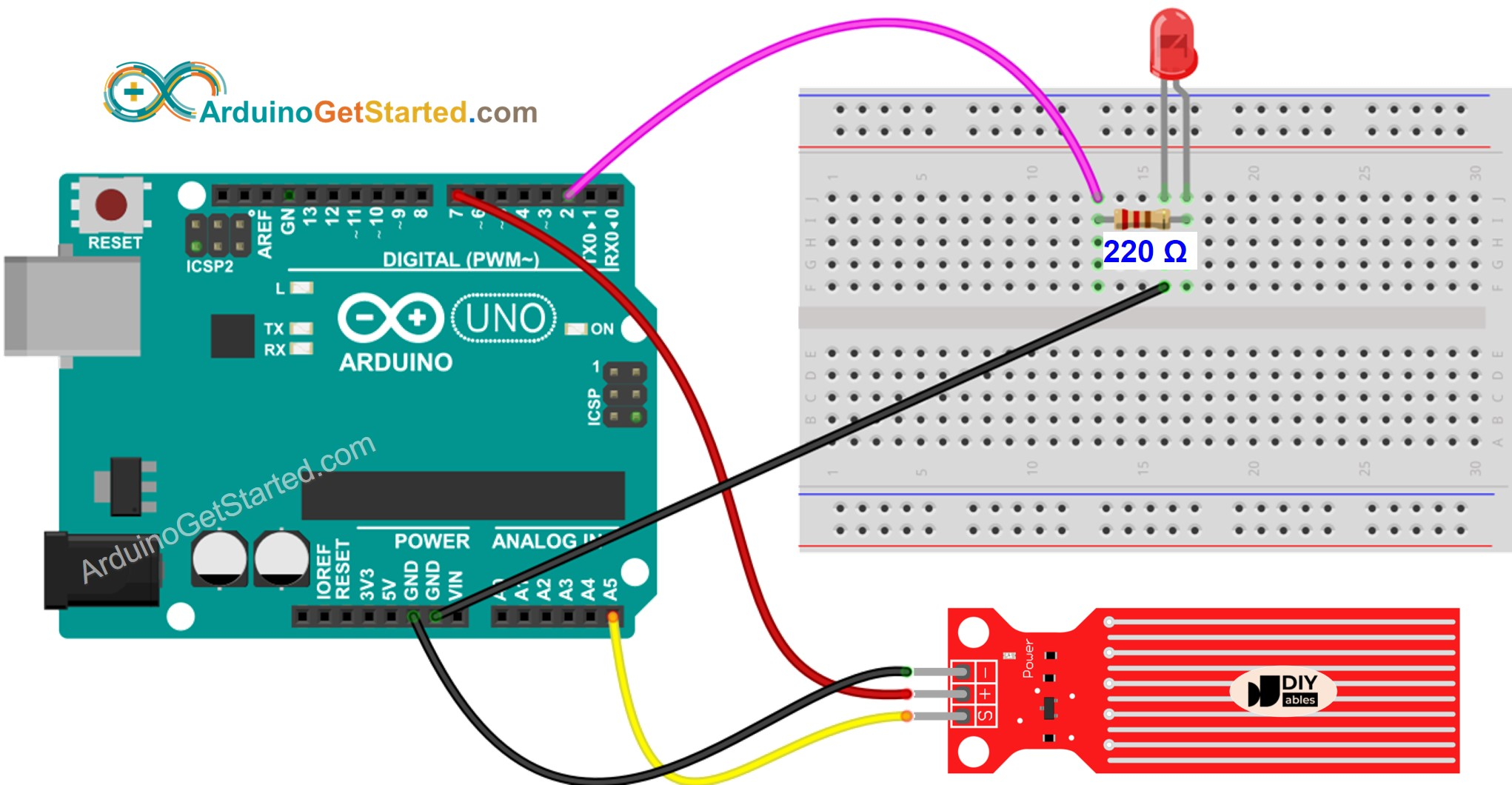# Arduino - Water Sensor

The water sensor or water level sensor is used to detect water leakage, rainfall, tank overflow, or to measure the water level. In this tutorial, we will learn:

• How to use the water sensor with Arduino
• How to connect the water sensor to Arduino
• How to program Arduino to read the state from the water sensor
• How to detect the water leakage, rainfall, tank overflow
• How to measure the water level
• How to calibrate the water sensor

## Hardware Required

Or you can buy the following sensor kit:

Please note: These are Amazon affiliate links. If you buy the components through these links, We will get a commission at no extra cost to you. We appreciate it.

### Water Level Sensor Pinout

The water level sensor has 3 pins:

• S (Signal) pin: is an analog output that will be connected to one of the analog inputs on your Arduino.
• + (VCC) pin: supplies power for the sensor. It is recommended to power the sensor with between 3.3V – 5V.
• - (GND) pin: is a ground connection.※ NOTE THAT:

The analog output value on the signal pin varies depending on the voltage supplied to the VCC pin of the sensor.

### How Water Level Sensor Works

Simply, The more water the sensor is immersed in, the higher the output voltage in the signal pin is.

Let's see more deeply.

This section is the in-depth knowledge. DON'T worry if you don't understand. Ignore this section if it overloads you, and come back in another day. Keep reading the next sections.

The sensor has a series of ten exposed copper traces. Five are power traces and five are sense traces. These traces are interlaced in parallel so that there is one sense trace between every two power traces. These traces are not connected unless they are bridged by water when submerged.

The traces act as a variable resistor (just like a potentiometer) whose resistance varies according to the water level.

• The change in resistance corresponds to the distance from the top of the sensor to the surface of the water.
• The resistance is inversely proportional to the height of the water:
• The more water the sensor is immersed in, the better the conductivity is, the lower the resistance is.
• The less water the sensor is immersed in, the worse the conductivity is, the higher the resistance is.
• The sensor produces an output voltage according to the resistance.

By measuring the voltage, we can determine the water level.

## Wiring Diagram

In theory, To supply power to the sensor, we can connect the sensor's VCC and GND pins to Arduino's 5v and GND pins, respectively.

In practice, however, that way is not recommended. Because in the moist environment, if we provide power to the sensor constantly, the sensor is electrochemically corroded faster. This shortens the sensor's lifespan. To overcome this issue, we recommend you to do not power the sensor all the time, but power the sensor only when reading the sensor's value. This can be done by connecting the sensor's VCC pin to a digital pin of an Arduino, and set the Arduino's pin to HIGH and LOW before and after reading, respectively.This image is created using Fritzing. Click to enlarge image

## Arduino Code - Reading Value from Water Sensor

/* * Created by ArduinoGetStarted.com * * This example code is in the public domain * * Tutorial page: https://arduinogetstarted.com/tutorials/arduino-water-sensor */ #define POWER_PIN 7 #define SIGNAL_PIN A5 int value = 0; // variable to store the sensor value void setup() { Serial.begin(9600); pinMode(POWER_PIN, OUTPUT); // configure D7 pin as an OUTPUT digitalWrite(POWER_PIN, LOW); // turn the sensor OFF } void loop() { digitalWrite(POWER_PIN, HIGH); // turn the sensor ON delay(10); // wait 10 milliseconds value = analogRead(SIGNAL_PIN); // read the analog value from sensor digitalWrite(POWER_PIN, LOW); // turn the sensor OFF Serial.print("Sensor value: "); Serial.println(value); delay(1000); }

### Quick Steps

• Copy the above code and open with Arduino IDE
• Click Upload button on Arduino IDE to upload code to Arduino
• Slowly dip the sensor into the water ( a glass of water).
• See the result on Serial Monitor. The value 0 when the sensor is not touching anything.
COM6
Sensor value: 0 Sensor value: 0 Sensor value: 0 Sensor value: 25 Sensor value: 97 Sensor value: 284 Sensor value: 428 Sensor value: 435 Sensor value: 441 Sensor value: 455 Sensor value: 467 Sensor value: 521 Sensor value: 528 Sensor value: 553
Autoscroll Show timestamp
Clear output
9600 baud
Newline

※ NOTE THAT:

The sensor is not designed to be fully submersed, only the exposed traces on the PCB can contact with water. Please be careful to install it.

## How To Detect Water Leakage

To detect the water leakage, rainfall, and tank overflow, we just need to compare the reading value with a threshold value. The threshold is determined in the calibration part of this tutorial.

Let's take a specific example. Arduino turns on an LED if the water is detected.

### Wiring DiagramThis image is created using Fritzing. Click to enlarge image

### Arduino Code - Detecting Water Leakage

/* * Created by ArduinoGetStarted.com * * This example code is in the public domain * * Tutorial page: https://arduinogetstarted.com/tutorials/arduino-water-sensor */ #define LED_PIN 2 #define POWER_PIN 7 #define SIGNAL_PIN A5 #define THRESHOLD 300 int value = 0; // variable to store the sensor value void setup() { Serial.begin(9600); pinMode(LED_PIN, OUTPUT); // configure D2 pin as an OUTPUT pinMode(POWER_PIN, OUTPUT); // configure D7 pin as an OUTPUT digitalWrite(POWER_PIN, LOW); // turn the sensor OFF digitalWrite(LED_PIN, LOW); // turn LED OFF } void loop() { digitalWrite(POWER_PIN, HIGH); // turn the sensor ON delay(10); // wait 10 milliseconds value = analogRead(SIGNAL_PIN); // read the analog value from sensor digitalWrite(POWER_PIN, LOW); // turn the sensor OFF if (value > THRESHOLD) { Serial.print("The water is detected"); digitalWrite(LED_PIN, HIGH); // turn LED ON } else { digitalWrite(LED_PIN, LOW); // turn LED OFF } }

## How To Measure The Water Level

If you want to divide the maximum height of water into some levels and measure the current level, you can use the method in the below code. Note that the maximum height of the water is equal to the height of the sensor. The below code divides the maximum height into 4 levels.

/* * Created by ArduinoGetStarted.com * * This example code is in the public domain * * Tutorial page: https://arduinogetstarted.com/tutorials/arduino-water-sensor */ #define POWER_PIN 7 #define SIGNAL_PIN A5 #define SENSOR_MIN 0 #define SENSOR_MAX 521 int value = 0; // variable to store the sensor value int level = 0; // variable to store the water level void setup() { Serial.begin(9600); pinMode(POWER_PIN, OUTPUT); // configure D7 pin as an OUTPUT digitalWrite(POWER_PIN, LOW); // turn the sensor OFF } void loop() { digitalWrite(POWER_PIN, HIGH); // turn the sensor ON delay(10); // wait 10 milliseconds value = analogRead(SIGNAL_PIN); // read the analog value from sensor digitalWrite(POWER_PIN, LOW); // turn the sensor OFF level = map(value, SENSOR_MIN, SENSOR_MAX, 0, 4); // 4 levels Serial.print("Water level: "); Serial.println(level); delay(1000); }

※ NOTE THAT:

• SENSOR_MIN and SENSOR_MAX is determined by the calibration process.
• The above mapping method is not accurate. However, it is acceptable in many applications. If you want to make it accurate, you can measure the threshold values for each level. See the calibration part

## Water Level Sensor Calibration

The output value of the sensor depends on not only the water level but also the water's conductivity. The pure water is not conductive. The water contains minerals and impurities is conductive. The more conductive the water is, the more sensitive the sensor is. On the other hand, the output value also varies depending on the voltage supplied to the VCC pin of the sensor.

To get accurate in reading the water sensor, We recommend you to calibrate the sensor for the particular type of water that you plan to monitor.

Before determining the threshold for triggering an action, you should measure the actual value read from the sensor by doing a test.

How to do the test:

• Use the above sketch for reading sensor value.
• Dip the sensor in the water at the level that you want it to be a threshold.
• Write down the value that the sensor outputs in Serial Monitor.
• Use the value as threshold you intend to trigger an action.

This test may take some trial and error.

The test can also be used to find:

• SENSOR_MIN value, when the sensor is not immersed in the water
• SENSOR_MAX value, when the sensor is fully immersed in the water
• A threshold value for detecting water leakage
• The threshold values for each level of your degree scale.

## Video Tutorial

We are considering to make the video tutorials. If you think the video tutorials are essential, please subscribe to our YouTube channel to give us motivation for making the videos.

## Challenge Yourself

• Send an email when detecting water leakage
• Send a SMS message when detecting water leakage
• Make a sound alarm when detecting water leakage

※ OUR MESSAGES